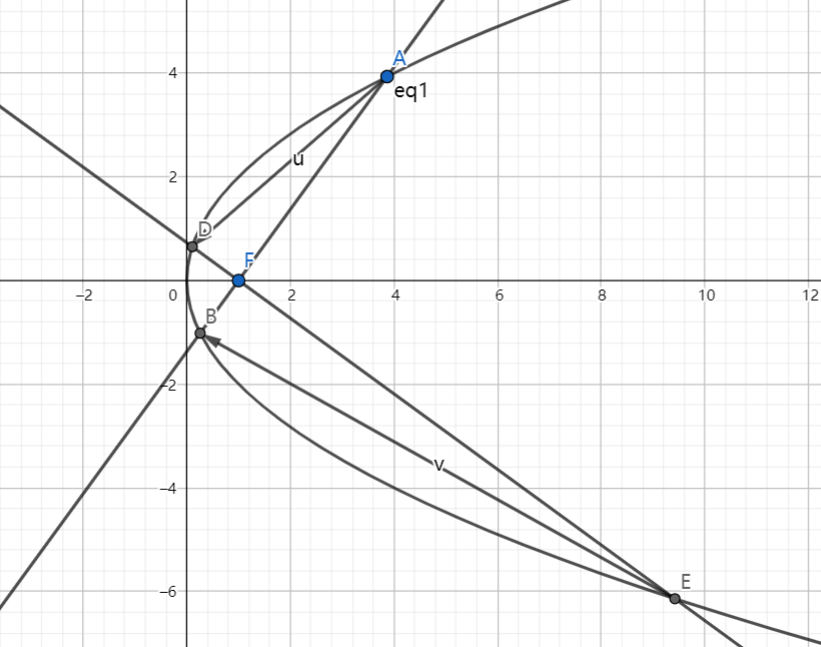# SAT1000 - P807

Algebra Level pendingAs shown above, parabola $C$ has equation: $y^2=4x$ and its focus is $F$.

Line $l_1, l_2$ both passes through $F$, $l_1$ intersects with $C$ at $A,B$, $l_2$ intersects with $C$ at $D,E$, $l_1 \perp l_2$.

Then find the minimum value of $\overrightarrow{AD} \cdot \overrightarrow{EB}$.

Let $M$ be the minimum value. Submit $\lfloor 1000M \rfloor$.

Have a look at my problem set: SAT 1000 problems

×